## Square numbers and square roots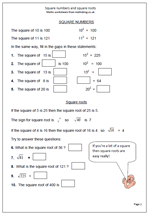A square number is a whole number multiplied by itself for example 3 x 3 = 9. This makes 9 a square number. By Year 6 most children will be familiar with square numbers and hopefully will know the first ten or so square numbers off be heart as they all appear in the times tables.

The square root of a number is a value, or number, that can be multiplied by itself to give the original number.

For example: the square root of 16 is 4 because 4 x 4 = 16

The square root of a number is usually written using the ‘tick’ square root sign. Each whole number is the square root of another number:

5 is the square root of 25

6 is the square root of 36 etc

This page takes a quick look at square numbers and square roots and can be found in our Year 6, Knowing Number facts section.

Square numbers and square roots

## Resource of the week: year 5 square numbers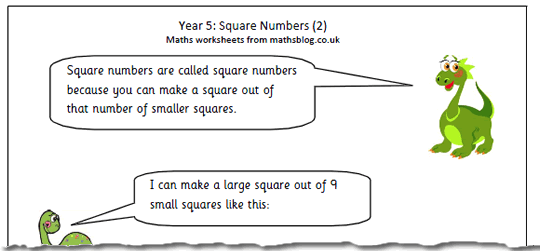This is just one of a short series of square number maths worksheets for Year 5.  It is an interesting and worthwhile exercise asking children to see if they can make a square out of 10 or 12 smaller squares (not overlapping etc) using plastic or card squares. Rectangles are possible, but not squares.

They can then be asked to find which numbers can be made into a square. This can be done either with smaller squares or as dots in an array.

There are several ways that questions involving square numbers can be phrased, including:

What is 4 squared?

What is the square of 4?

What number multiplied by itself makes 16?

Square numbers 2

## Resource of the week: Tables square and square numbers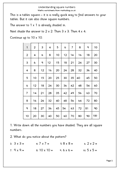This worksheet has a 10×10 multiplication square which is a brilliant aid to helping with tables, but it is also excellent for showing the pattern of square numbers from 1 to 10.

A square number is made by multiplying a whole number by itself. eg 4 x 4 = 16.

16 is a square number.

It is quite useful to learn the first 10 square numbers off by heart:

1, 4, 9, 16, 25, 36, 49, 64, 81, 100.

We will look more closely at square numbers later.

Square numbers (pg 1)

## Year 6 Maths worksheet: Square numbers (2)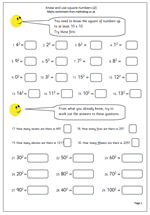Here we have a follow up page for the year 6 maths worksheet on square numbers published on Thursday. Further practice at remembering square numbers and using this knowledge to work out square numbers of multiples of 10.

Know and use square numbers (2)

## Year 6: Know and use square numbers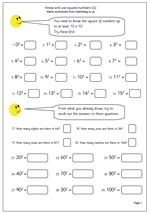By Year 6 children are expected to know the square of numbers up to at least 10. This page provides some revision of this together with some extension work on finding square numbers of multiples of 10. Care needs to be taken when finding these. For example, because 50 is 5 x 10, the square of 50 can be broken down into 5 x 5 x 10 x 10 which makes 250 x10 which is 2 500. Keep an eye out for some children who think it is just 250.

This page can be found in our Year 6, Knowing number facts section. It is also a useful page for the year 6 planning: Block B, Unit 3, week 2 for those teacher viewers.

Know and use square numbers (1)

## Year 5 Maths Worksheet: Square Numbers (2)This is the second in our series of square number maths worksheets for Year 5.  It is an interesting and worthwhile exercise asking children to see if they can make a square out of 10 or 12 smaller squares (not overlapping etc) using plastic or card squares. Rectangles are possible, but not squares.

They can then be asked to find which numbers can be made into a square. This can be done either with smaller squares or as dots in an array.

There are several ways that questions involving square numbers can be phrased, including:

What is 4 squared?

What is the square of 4?

What number multiplied by itself makes 16?

Square numbers 2

## Times tables and square numbers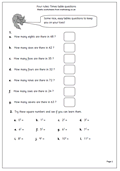Rather a mixed bag of questions here. The first half asks questions derived from the times tables, such as ‘How many fours in 32?’ This is an important question to ask before moving on to the long method of division.

The second set of questions looks at square numbers, which, of course, give a diagonal pattern on a tables square. Children need to be familiar with the square sign to tackle this. It is extremely useful to know, off by heart, all the square numbers, up to at least 10 x 10, as it will be a great help later in High School.

This page can be found in our Four Rules section, under Multiplication (mental methods).

Times table questions (pg 1)

## Year 5 maths worksheet: square numbers 1This worksheet has a 10×10 multiplication square which is a brilliant aid to helping with tables, but it is also excellent for showing the pattern of square numbers from 1 to 10.

A square number is made by multiplying a whole number by itself. eg 4 x 4 = 16.

16 is a square number.

It is quite useful to learn the first 10 square numbers off by heart:

1, 4, 9, 16, 25, 36, 49, 64, 81, 100.

We will look more closely at square numbers later.

Square numbers (pg 1)